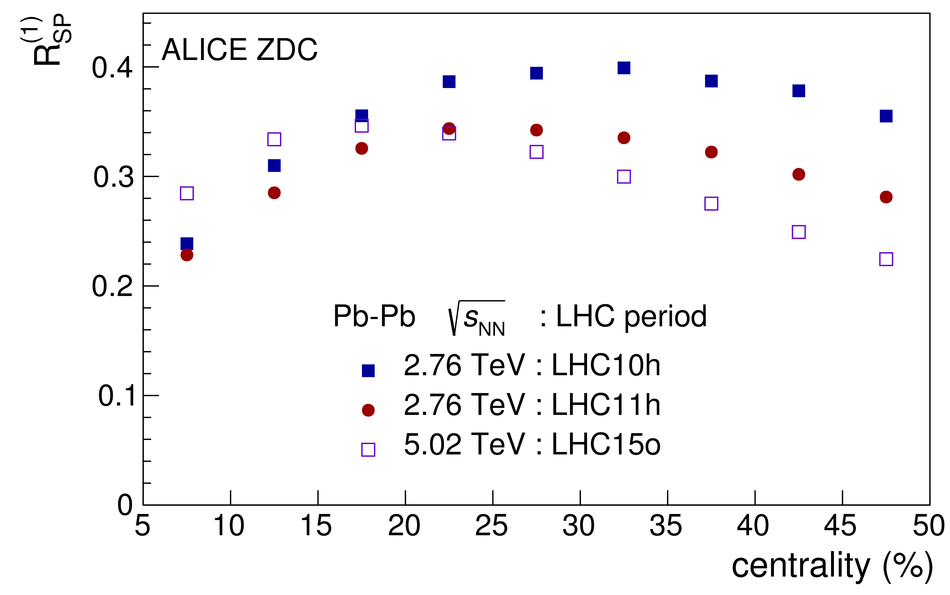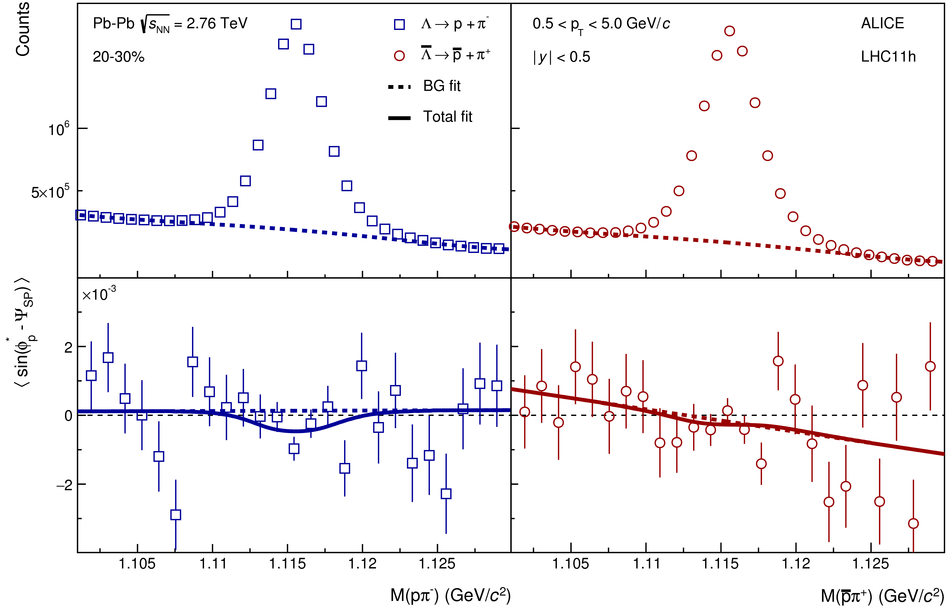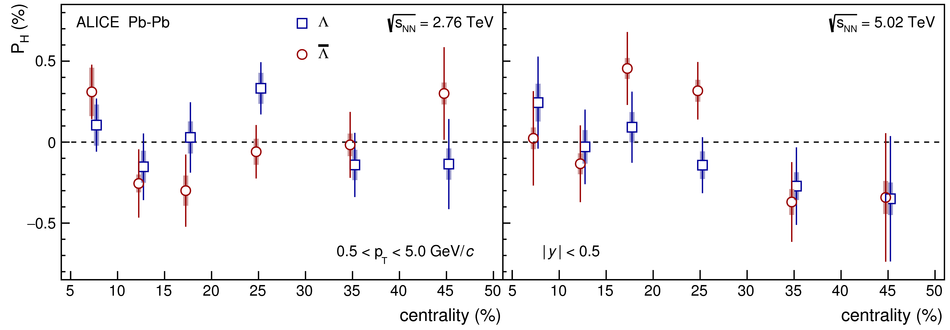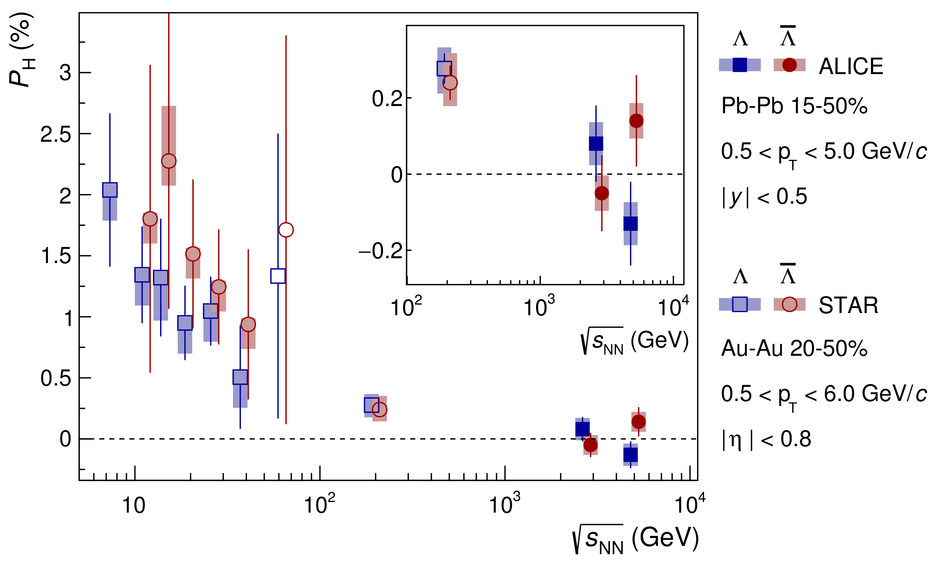# Global polarization of $Λ$ and $\overlineΛ$ hyperons in Pb-Pb collisions at the LHC

The global polarization of the $\Lambda$ and $\overline\Lambda$ hyperons is measured for Pb-Pb collisions at $\sqrt{s_{\rm{NN}}}$ = 2.76 and 5.02 TeV recorded with the ALICE at the LHC. The results are reported differentially as a function of collision centrality and hyperon's transverse momentum ($p_{\rm{T}}$) for the range of centrality 5-50%, $0.5 <~ p_{\rm{T}} <~5$ GeV/$c$, and rapidity $|y|<~0.5$. The hyperon global polarization averaged for Pb-Pb collisions at $\sqrt{s_{\rm{NN}}}$ = 2.76 and 5.02 TeV is found to be consistent with zero, $\langle P_{\rm{H}}\rangle$ (%) $\approx$ 0.01 $\pm$ 0.06 (stat.) $\pm$ 0.03 (syst.) in the collision centrality range 15-50%, where the largest signal is expected. The results are compatible with expectations based on an extrapolation from measurements at lower collision energies at RHIC, hydrodynamical model calculations, and empirical estimates based on collision energy dependence of directed flow, all of which predict the global polarization values at LHC energies of the order of 0.01%.

Figures

## Figure 1

 The correction $\Rep$ for finite resolution of the spectator plane angle $\psisp$ as a function of collision centrality for three data sets used in the analysis Only statistical uncertainties, which are smaller than a symbol size, are shown## Figure 2

 (Upper panels) invariant mass distributions of $\Lambda$ (left) and $\overline\Lambda$ (right) candidates for 20--30\% centrality range in Pb--Pb collisions at $\sNN=2.76$~TeV using data collected during the LHC operation in 2011 Dashed lines show the result of the fit for $N_{\rm BG}(\Minv)$ in Eq.~(\ref{Eq:fBG}) (Bottom panels) Global polarization extraction via fit to $\left< \sin(\varphi^*_{\rm p}-\psisp)\right>$ as a function of the invariant mass, with $\psisp$ reconstructed with the ZDC on the target ($\eta< 0$) side Dashed and solid lines show $L_\mathrm{BG}(\Minv)$ and the combined fit with Eq.~(\ref{eq:SinFit}) See text for more details## Figure 3

 The global hyperon polarization as function of centrality for Pb--Pb collisions at $\sNN=2.76$~TeV (left) and $5.02$~TeV (right) The systematic uncertainties are shown as shaded boxes Points are slightly shifted along the horizontal axis for better visibility## Figure 4

 The global hyperon polarization as function of transverse momentum \pT for Pb--Pb collisions at $\sNN=2.76$~TeV (upper) and $5.02$~TeV (lower) in 5--15\% (left) and 15--50\% (right) centrality classes The systematic uncertainties are shown as shaded boxes Points are slightly shifted along the horizontal axis for better visibility## Figure 5

 The global hyperon polarization as a function of collision energy Results are compared with the STAR data at lower energies~ The insert shows zoomed-in comparison with the data at the top RHIC energy The systematic uncertainties are shown as shaded boxes Points are slightly shifted along the horizontal axis for better visibility